### Home > CALC > Chapter Ch2 > Lesson 2.3.3 > Problem2-131

2-131.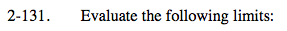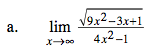This is a limit as x→∞, so we are checking to see if there is a horizontal asymptote, or not.

We only need to consider the highest power of the numerator with the highest power of the denominator.

$\text{Highest power in the numerator: }\sqrt{9x^{2}}=3x$

$\text{Highest power in the denominator: }4x^{2}$

$=\lim_{x \rightarrow \infty} \frac{3x}{4x^{2}}= \lim_{x \rightarrow \infty} \frac{3}{4x}=$

This is also the equation of the horizontal asymptote.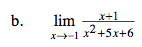Nothing elaborate here. Try just substituting in −1.

0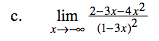Refer to the hints in part (a). But notice that we are evaluating limit as x→−∞, which also will reveal the equation of a horizontal asymptote, if there is one.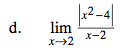Find both the limit from the left and the limit from the right. Then compare them.

If the limit exists, then there must be agreement from the left and the right AND that limit must be finite.